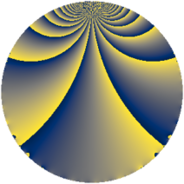# Properties

 Label 1560.1.yLevel $1560$ Weight $1$ Character orbit 1560.y Rep. character $\chi_{1560}(389,\cdot)$ Character field $\Q$ Dimension $8$ Newform subspaces $6$ Sturm bound $336$ Trace bound $3$

# Related objects

## Defining parameters

 Level: $$N$$ $$=$$ $$1560 = 2^{3} \cdot 3 \cdot 5 \cdot 13$$ Weight: $$k$$ $$=$$ $$1$$ Character orbit: $$[\chi]$$ $$=$$ 1560.y (of order $$2$$ and degree $$1$$) Character conductor: $$\operatorname{cond}(\chi)$$ $$=$$ $$1560$$ Character field: $$\Q$$ Newform subspaces: $$6$$ Sturm bound: $$336$$ Trace bound: $$3$$

## Dimensions

The following table gives the dimensions of various subspaces of $$M_{1}(1560, [\chi])$$.

Total New Old
Modular forms 16 16 0
Cusp forms 8 8 0
Eisenstein series 8 8 0

The following table gives the dimensions of subspaces with specified projective image type.

$$D_n$$ $$A_4$$ $$S_4$$ $$A_5$$
Dimension 8 0 0 0

## Trace form

 $$8 q + 8 q^{9} + O(q^{10})$$ $$8 q + 8 q^{9} - 4 q^{10} + 8 q^{16} + 4 q^{30} - 8 q^{39} - 4 q^{40} - 8 q^{49} - 8 q^{55} + 8 q^{66} + 8 q^{81} - 4 q^{90} - 8 q^{94} + O(q^{100})$$

## Decomposition of $$S_{1}^{\mathrm{new}}(1560, [\chi])$$ into newform subspaces

Label Dim $A$ Field Image CM RM Traces $q$-expansion
$a_{2}$ $a_{3}$ $a_{5}$ $a_{7}$
1560.1.y.a $1$ $0.779$ $$\Q$$ $D_{2}$ $$\Q(\sqrt{-39})$$, $$\Q(\sqrt{-390})$$ $$\Q(\sqrt{10})$$ $$-1$$ $$-1$$ $$1$$ $$0$$ $$q-q^{2}-q^{3}+q^{4}+q^{5}+q^{6}-q^{8}+\cdots$$
1560.1.y.b $1$ $0.779$ $$\Q$$ $D_{2}$ $$\Q(\sqrt{-39})$$, $$\Q(\sqrt{-390})$$ $$\Q(\sqrt{10})$$ $$-1$$ $$1$$ $$1$$ $$0$$ $$q-q^{2}+q^{3}+q^{4}+q^{5}-q^{6}-q^{8}+\cdots$$
1560.1.y.c $1$ $0.779$ $$\Q$$ $D_{2}$ $$\Q(\sqrt{-39})$$, $$\Q(\sqrt{-390})$$ $$\Q(\sqrt{10})$$ $$1$$ $$-1$$ $$-1$$ $$0$$ $$q+q^{2}-q^{3}+q^{4}-q^{5}-q^{6}+q^{8}+\cdots$$
1560.1.y.d $1$ $0.779$ $$\Q$$ $D_{2}$ $$\Q(\sqrt{-39})$$, $$\Q(\sqrt{-390})$$ $$\Q(\sqrt{10})$$ $$1$$ $$1$$ $$-1$$ $$0$$ $$q+q^{2}+q^{3}+q^{4}-q^{5}+q^{6}+q^{8}+\cdots$$
1560.1.y.e $2$ $0.779$ $$\Q(\sqrt{-1})$$ $D_{2}$ $$\Q(\sqrt{-39})$$, $$\Q(\sqrt{-30})$$ $$\Q(\sqrt{130})$$ $$0$$ $$-2$$ $$0$$ $$0$$ $$q-iq^{2}-q^{3}-q^{4}-iq^{5}+iq^{6}+iq^{8}+\cdots$$
1560.1.y.f $2$ $0.779$ $$\Q(\sqrt{-1})$$ $D_{2}$ $$\Q(\sqrt{-39})$$, $$\Q(\sqrt{-30})$$ $$\Q(\sqrt{130})$$ $$0$$ $$2$$ $$0$$ $$0$$ $$q-iq^{2}+q^{3}-q^{4}+iq^{5}-iq^{6}+iq^{8}+\cdots$$